### Review of single and multi-degree of freedom (mdof) systems: Center of Percussion and Vibration of a Compound Pendulum

The concept of the center of percussion is usually discussed using the analysis of the changes in angular momentum and linear momentum associated with a body impacting a rotating object and essentially determining the point at which there is no change in acceleration at the pivot point due to this impact. (The usual example is a ball hitting a baseball or cricket bat.) For the compound pendulum shown the natural frequency is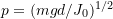where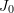is the moment of inertia of the body about the axis of rotation at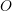. Instead of having a distributed mass, let the mass of the pendulum be concentrated at a single point located at a distance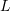from the pivot point. The natural frequency of this simple pendulum is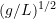. If the natural frequencies of these two pendulums are the same, then the point at whichis concentrated is called the center of oscillation and is identical to the center of percussion. If instead, we consider rotation about the center of oscillation (percussion) then the original pivot atbecomes the center of percussion (oscillation).

Early automobile manufacturers did not realize this and as a result, cars had much more uncomfortable rides because impacts at one set of wheels (front or rear) were felt at the other. One of the first automobile designs to incorporate this idea was the very futuristic Chysler Airflow (circa 1930) which was years ahead in its design in a number of aspects. (So far ahead that it was a commercial failure.) For the illustration of the automobile shown the relationship between the position of the center of gravity and the positions of the front and rear wheels is given by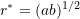where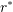is the radius of gyration of the vehicle about its center of gravity and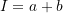is the wheelbase (andare the horizontal distances from the front and rear wheels to the center of gravity). From a vibration perspective if a car is designed with a mass distribution to meet these criteria (almost all recent automobiles are) then we can examine what happens at one set of wheels individually for certain situations (e.g. hitting a bump with the front wheels only).

Using the diagram below show that the condition for the center of percussion to be at the front wheels with the pivot at the rear wheels that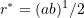and that this is also true for the pivot point moving to the front wheels with the impact at the rear ones.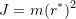Where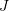is the moment of inertia about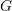andis the radius of gyration about: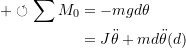Therefore: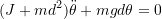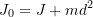Therefore: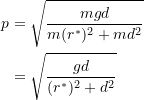#### Equivalent Pendulum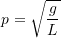Therefore choose: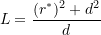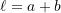For application to the auto above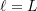(wheelbase). For rotation about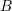: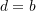Therefore: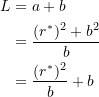Therefore: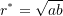For rotation about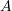: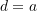Therefore: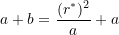#### Determination of Damping Ratio

We know that for any SDOF viscously damped system the equation for free vibration is: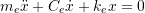and the system parameters are the natural frequency: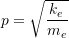and the damping ratio: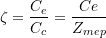We can determine the damping ratio from how rapidly the vibration decays. To do this we use the so-called logarithmic decrement. Consider the damped response: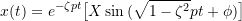which for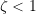looks like:

These peaks occur approximately when: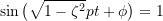Therefore if the first occurs at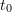then the next time it is at: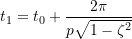Therefore: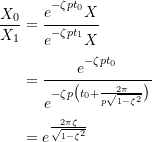and this is the same ratio for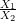,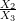, etc.

Therefore this creates the logarithmic decrement equation: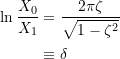If we take n cycles: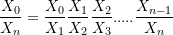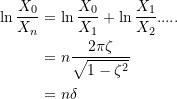Therefore: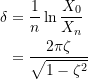Therefore if we measure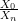we can easily determine the damping ratio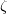.

If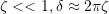It does not take a largeto quickly damp out vibrations.